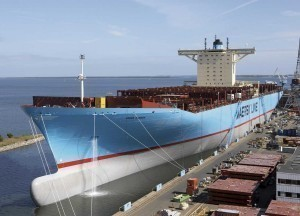# Worlds Biggest Cargo Ship

The world’s biggest cargo ship is the Emma Maersk. It has a maximum TEU of 15,200. It is 397.7 m long with a beam of 56.4 m. The Emma Maersk has six sister ships of the same size. The GT of the ships are 151,687.

### The Largest Cargo Ships from 2 to 5

The second largest is the MSC Danit with a 14,000 TEU. It is a 365.50 m long with a beam measuring 51.20 m. The GT is 153,092. The MSC Beatrice also has a maximum TEU of 14,000. However, its GT is only 151,559. The ship is 366 m long and has a beam of 51 m.

At number three is the CMA CGM Thalassa with a 10,960 maximum TEU. The GT is 128,600 and the length is 346.5 m. The beam is 45.6 m.

At number four is Gudrun Maersk with a maximum TEU of 10,150. It is 367.3 m long and with a beam of 42.8 m. The GT is 97,933. The Clementine Maersk is at number five. The maximum TEU is 9,600. The length is 348.7 and the beam is 42.6 m. The GT is 96000.

### The World’s Biggest Cargo Ships: Number Six to NineThe COSCO Guangzhou is at number six. It has a maximum TEU of 9,450 and the GT is 99,833. The length is 350 m and the beam is 42.8 m. The CMA CGM Medea is at number seven. It has a maximum TEU of 9,415 and the GT is 99,500. The ship is 350 m long and the beam is 42.8 m.

The Axel Maersk is at number eight. It has a maximum TEU of 9,310. The GT is 93,496 and the length is 352.6 m. The beam is 42.8 m. At number nine is the NYK Vega. It is 338.2 m long and the beam is 45.6 m. The maximum TEU is 9,200 and the GT is 97,825.

### TEU

TEU stands for twenty-foot equivalent unit. This is the capacity used for all cargo and container ships. The typical 20 foot container measures 20 × 8.0 × 8.5 feet (6.1 × 2.4 × 2.6 meters). Even so, majority of ships today use 40 ft long containers.

There is no telling how long the Emma Maersk will remain the world’s biggest cargo ship. Because of the need to transport large volumes of cargoes, the sizes of the ships are expected to grow too.